# Remapping¶

## Goal¶

In this tutorial you will learn how to:

1. Use the OpenCV function remap to implement simple remapping routines.

## Theory¶

### What is remapping?¶

• It is the process of taking pixels from one place in the image and locating them in another position in a new image.

• To accomplish the mapping process, it might be necessary to do some interpolation for non-integer pixel locations, since there will not always be a one-to-one-pixel correspondence between source and destination images.

• We can express the remap for every pixel location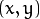as: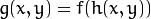where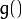is the remapped image,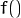the source image and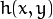is the mapping function that operates on.

• Let’s think in a quick example. Imagine that we have an imageand, say, we want to do a remap such that: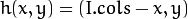What would happen? It is easily seen that the image would flip in the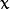direction. For instance, consider the input image: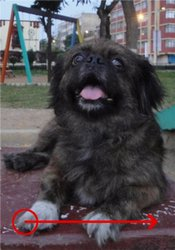observe how the red circle changes positions with respect to x (consideringthe horizontal direction):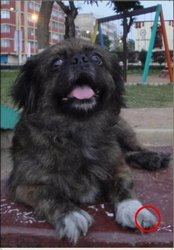• In OpenCV, the function remap offers a simple remapping implementation.

## Code¶

1. What does this program do?
• Loads an image
• Each second, apply 1 of 4 different remapping processes to the image and display them indefinitely in a window.
• Wait for the user to exit the program
2. The tutorial code’s is shown lines below. You can also download it from here
 #include "opencv2/highgui/highgui.hpp"
#include "opencv2/imgproc/imgproc.hpp"
#include <iostream>
#include <stdio.h>

using namespace cv;

/// Global variables
Mat src, dst;
Mat map_x, map_y;
char* remap_window = "Remap demo";
int ind = 0;

/// Function Headers
void update_map( void );

/**
* @function main
*/
int main( int argc, char** argv )
{
/// Load the image
src = imread( argv, 1 );

/// Create dst, map_x and map_y with the same size as src:
dst.create( src.size(), src.type() );
map_x.create( src.size(), CV_32FC1 );
map_y.create( src.size(), CV_32FC1 );

/// Create window
namedWindow( remap_window, CV_WINDOW_AUTOSIZE );

/// Loop
while( true )
{
/// Each 1 sec. Press ESC to exit the program
int c = waitKey( 1000 );

if( (char)c == 27 )
{ break; }

/// Update map_x & map_y. Then apply remap
update_map();
remap( src, dst, map_x, map_y, CV_INTER_LINEAR, BORDER_CONSTANT, Scalar(0,0, 0) );

/// Display results
imshow( remap_window, dst );
}
return 0;
}

/**
* @function update_map
* @brief Fill the map_x and map_y matrices with 4 types of mappings
*/
void update_map( void )
{
ind = ind%4;

for( int j = 0; j < src.rows; j++ )
{ for( int i = 0; i < src.cols; i++ )
{
switch( ind )
{
case 0:
if( i > src.cols*0.25 && i < src.cols*0.75 && j > src.rows*0.25 && j < src.rows*0.75 )
{
map_x.at<float>(j,i) = 2*( i - src.cols*0.25 ) + 0.5 ;
map_y.at<float>(j,i) = 2*( j - src.rows*0.25 ) + 0.5 ;
}
else
{ map_x.at<float>(j,i) = 0 ;
map_y.at<float>(j,i) = 0 ;
}
break;
case 1:
map_x.at<float>(j,i) = i ;
map_y.at<float>(j,i) = src.rows - j ;
break;
case 2:
map_x.at<float>(j,i) = src.cols - i ;
map_y.at<float>(j,i) = j ;
break;
case 3:
map_x.at<float>(j,i) = src.cols - i ;
map_y.at<float>(j,i) = src.rows - j ;
break;
} // end of switch
}
}
ind++;
}


## Explanation¶

1. Create some variables we will use:

Mat src, dst;
Mat map_x, map_y;
char* remap_window = "Remap demo";
int ind = 0;

2. Load an image:

src = imread( argv, 1 );

3. Create the destination image and the two mapping matrices (for x and y )

dst.create( src.size(), src.type() );
map_x.create( src.size(), CV_32FC1 );
map_y.create( src.size(), CV_32FC1 );

4. Create a window to display results

namedWindow( remap_window, CV_WINDOW_AUTOSIZE );

5. Establish a loop. Each 1000 ms we update our mapping matrices (mat_x and mat_y) and apply them to our source image:

while( true )
{
/// Each 1 sec. Press ESC to exit the program
int c = waitKey( 1000 );

if( (char)c == 27 )
{ break; }

/// Update map_x & map_y. Then apply remap
update_map();
remap( src, dst, map_x, map_y, CV_INTER_LINEAR, BORDER_CONSTANT, Scalar(0,0, 0) );

/// Display results
imshow( remap_window, dst );
}


The function that applies the remapping is remap. We give the following arguments:

• src: Source image
• dst: Destination image of same size as src
• map_x: The mapping function in the x direction. It is equivalent to the first component of• map_y: Same as above, but in y direction. Note that map_y and map_x are both of the same size as src
• CV_INTER_LINEAR: The type of interpolation to use for non-integer pixels. This is by default.
• BORDER_CONSTANT: Default

How do we update our mapping matrices mat_x and mat_y? Go on reading:

6. Updating the mapping matrices: We are going to perform 4 different mappings:

1. Reduce the picture to half its size and will display it in the middle: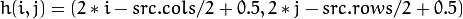for all pairs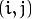such that: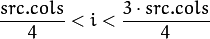and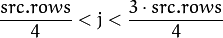2. Turn the image upside down: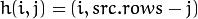3. Reflect the image from left to right: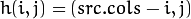4. Combination of b and c: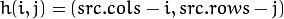This is expressed in the following snippet. Here, map_x represents the first coordinate of h(i,j) and map_y the second coordinate.

for( int j = 0; j < src.rows; j++ )
{ for( int i = 0; i < src.cols; i++ )
{
switch( ind )
{
case 0:
if( i > src.cols*0.25 && i < src.cols*0.75 && j > src.rows*0.25 && j < src.rows*0.75 )
{
map_x.at<float>(j,i) = 2*( i - src.cols*0.25 ) + 0.5 ;
map_y.at<float>(j,i) = 2*( j - src.rows*0.25 ) + 0.5 ;
}
else
{ map_x.at<float>(j,i) = 0 ;
map_y.at<float>(j,i) = 0 ;
}
break;
case 1:
map_x.at<float>(j,i) = i ;
map_y.at<float>(j,i) = src.rows - j ;
break;
case 2:
map_x.at<float>(j,i) = src.cols - i ;
map_y.at<float>(j,i) = j ;
break;
case 3:
map_x.at<float>(j,i) = src.cols - i ;
map_y.at<float>(j,i) = src.rows - j ;
break;
} // end of switch
}
}
ind++;
}


## Result¶

1. After compiling the code above, you can execute it giving as argument an image path. For instance, by using the following image: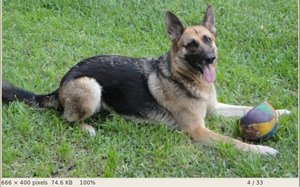2. This is the result of reducing it to half the size and centering it: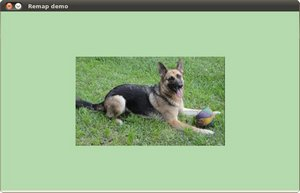3. Turning it upside down: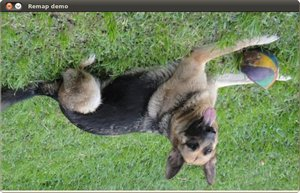4. Reflecting it in the x direction: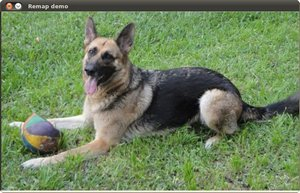5. Reflecting it in both directions: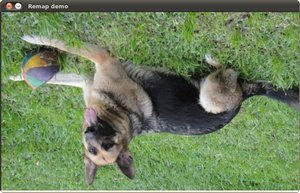### Table Of Contents

#### Previous topic

Hough Circle Transform

#### Next topic

Affine Transformations